Published

# CovidStop

A technology which increases efficiency in signing in or out during peak hour areas when entering the campus while the SafeEntry implies.

IntermediateWork in progress10 hours748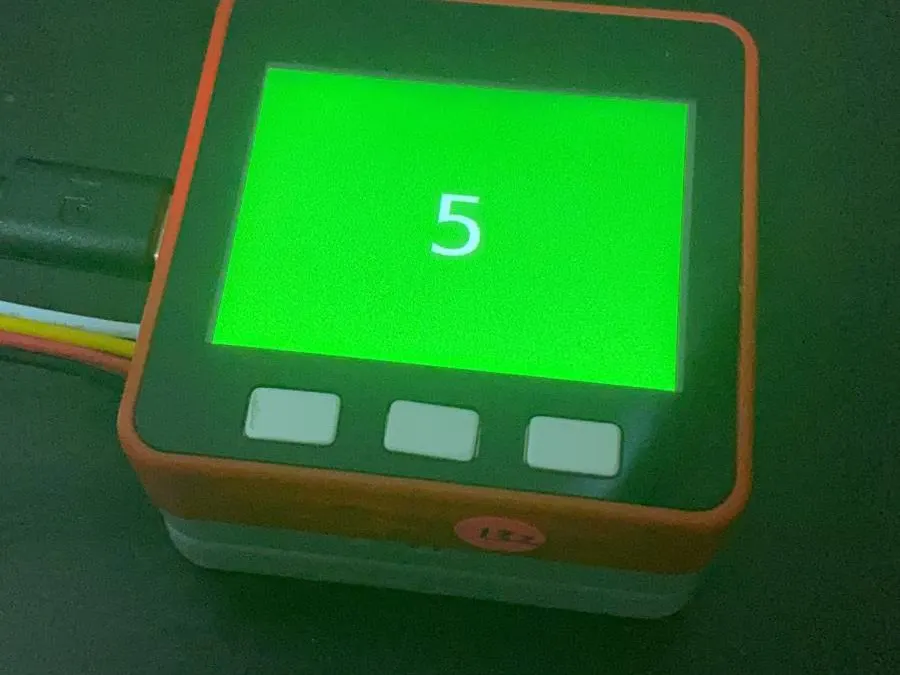## Things used in this project

### Hardware components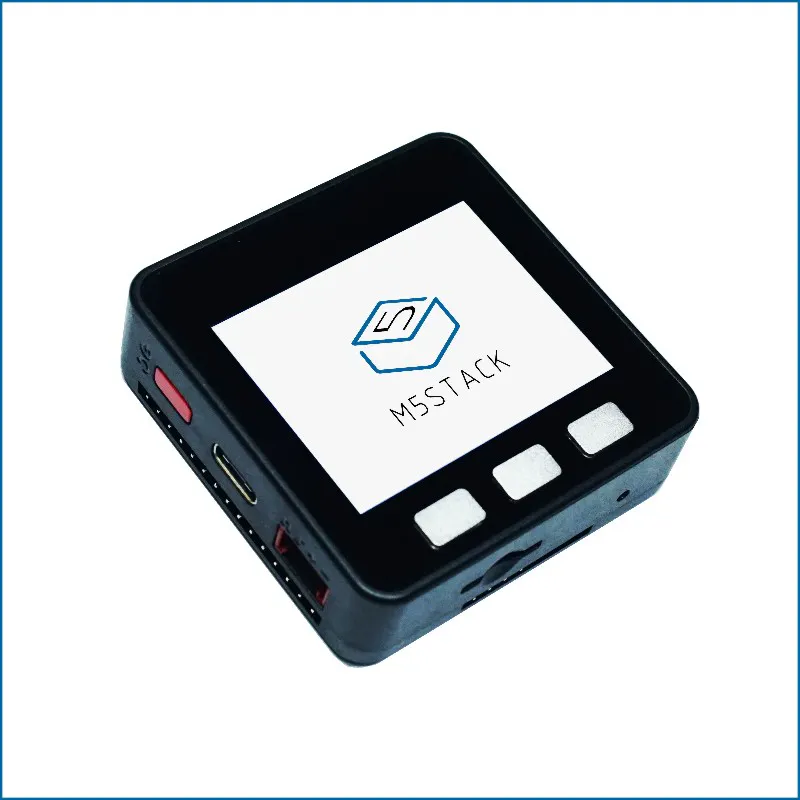M5Stack ESP32 Basic Core IoT Development Kit
×2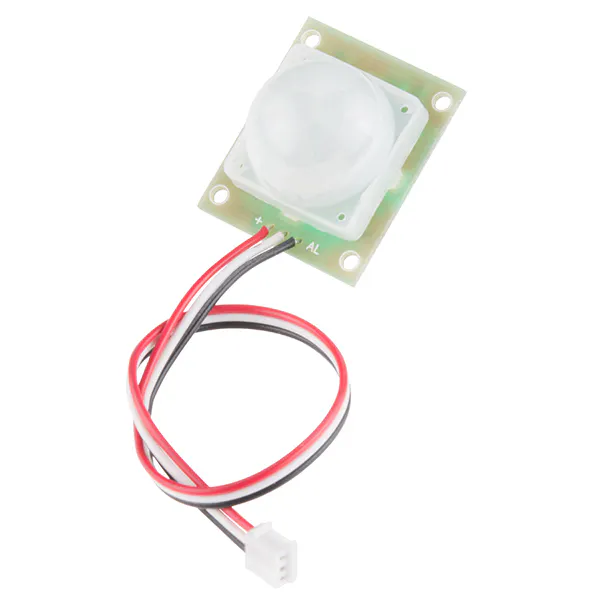PIR Motion Sensor (generic)
×1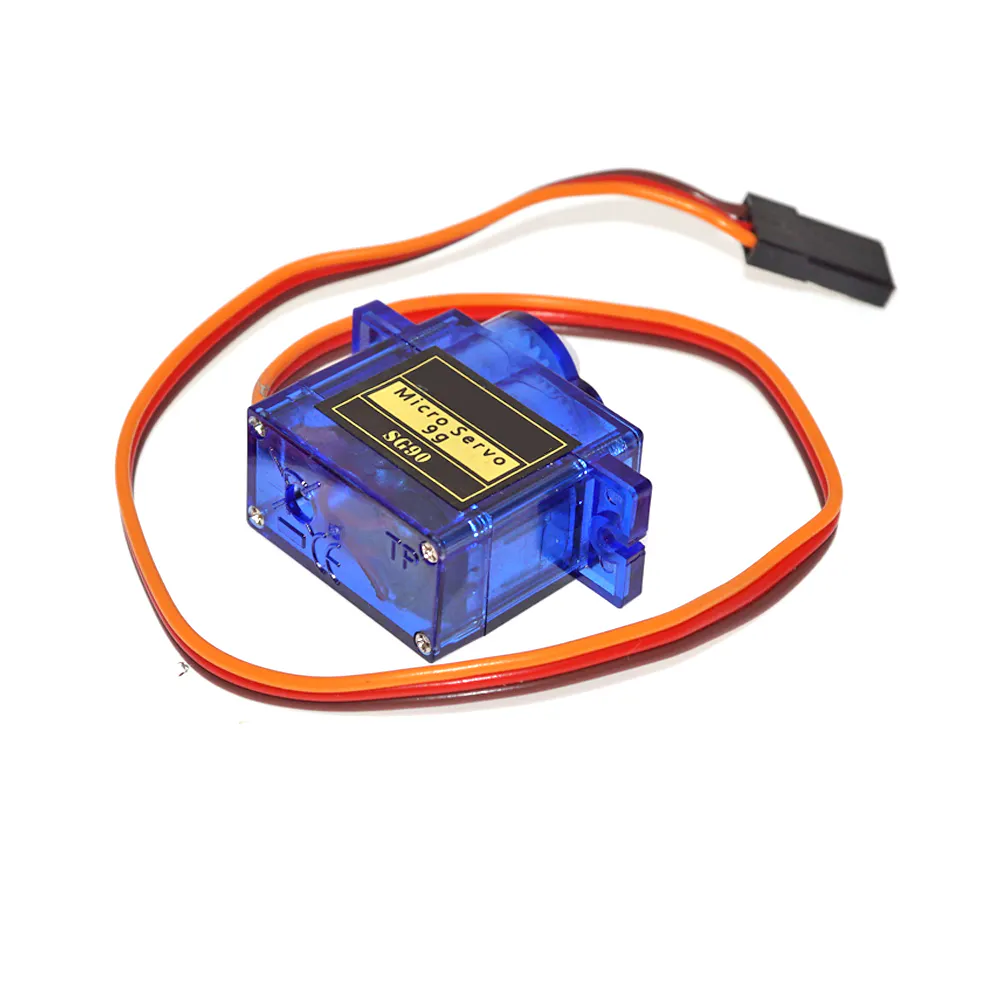SG90 Micro-servo motor
×1Hub, 3 Ports
×1
 RGB Unit
×1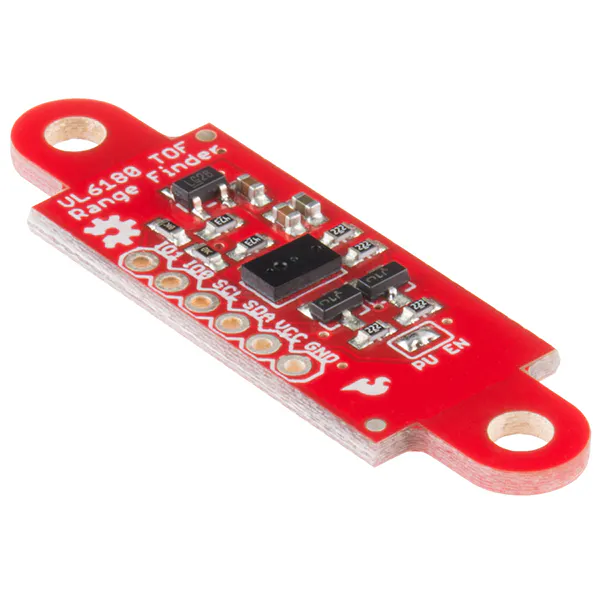SparkFun ToF Range Finder Sensor - VL6180
×1
 ENVii
×1
 RFID
×1

### Software apps and online services

 ui flow

## Schematics

### M5Stack A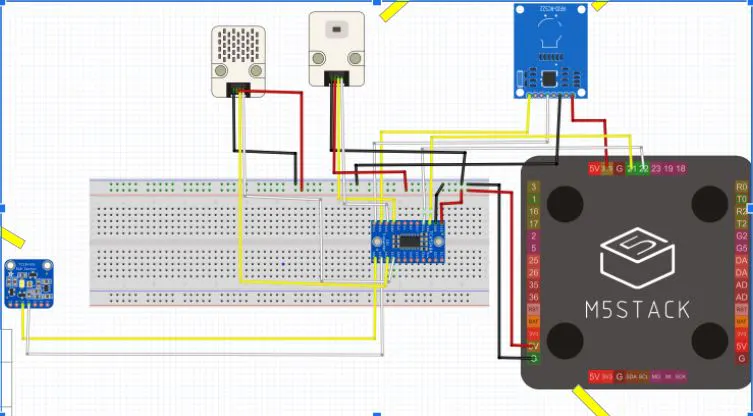### M5Stack B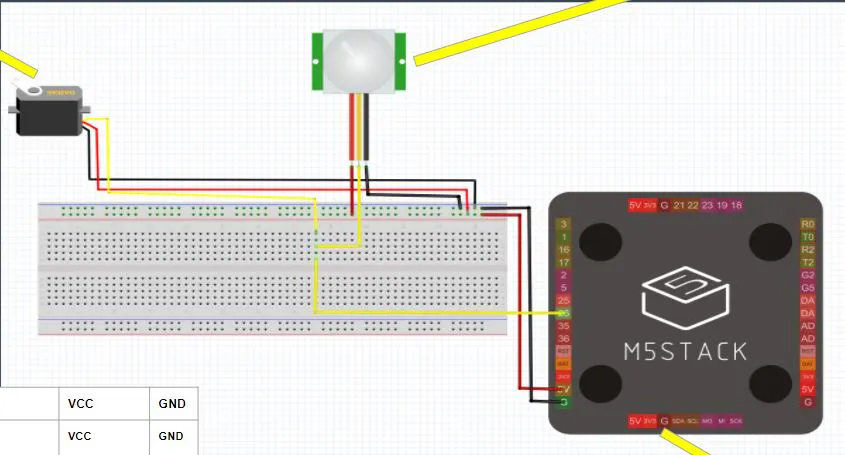## Code

### M5Stack A

Python
First part of the code; from the first m5stack
```from m5stack import *
from m5ui import *
from uiflow import *
import time
import unit

setScreenColor(0x000000)
rgb3 = unit.get(unit.RGB, unit.PORTB)
rfid2 = unit.get(unit.RFID, unit.PAHUB0)
pahub4 = unit.get(unit.PAHUB, unit.PORTA)
tof1 = unit.get(unit.TOF, unit.PAHUB1)
env27 = unit.get(unit.ENV2, unit.PAHUB2)

x = None

scannedpeople = M5TextBox(115, 105, "0", lcd.FONT_DejaVu72, 0xFFFFFF, rotate=0)
label0 = M5TextBox(24, 35, "Temperature:", lcd.FONT_DejaVu18, 0xFFFFFF, rotate=0)
label1 = M5TextBox(173, 40, "___", lcd.FONT_Default, 0xFFFFFF, rotate=0)
label2 = M5TextBox(124, 72, "___", lcd.FONT_Default, 0xFFFFFF, rotate=0)

x = 0
while True:
label1.setText(str(env27.temperature))
if (rfid2.isCardOn()) == True:
rgb3.setColor(1, 0x33ff33)
rgb3.setColor(2, 0x33ff33)
rgb3.setColor(3, 0x33ff33)
x = x + 1
speaker.tone(1000, 10)
scannedpeople.setFont(lcd.FONT_DejaVu72)
scannedpeople.setText(str(x))
wait(1)
elif (rfid2.isCardOn()) == False:
rgb3.setColor(1, 0xff0000)
rgb3.setColor(2, 0xff0000)
rgb3.setColor(3, 0xff0000)
scannedpeople.setText(str(x))
if (tof1.distance) < 200:
scannedpeople.setText('STAND BACK')
speaker.tone(10000, 10)
rgb3.setColor(1, 0xffffff)
rgb3.setColor(2, 0xffffff)
rgb3.setColor(3, 0xffffff)
else:
scannedpeople.setFont(lcd.FONT_DejaVu24)
wait(1)
if (env27.temperature) > 32:
speaker.tone(10000, 10)
elif (env27.temperature) < 30:
speaker.tone(10000, 10)
else:
label2.setText('Welcome')
wait_ms(2)
```

### M5Stack B

Python
Second part of the code; from the second m5stack
```from m5stack import *
from m5ui import *
from uiflow import *
import machine
import unit

setScreenColor(0x222222)
servo0 = unit.get(unit.SERVO, unit.PORTA)
pir1 = unit.get(unit.PIR, unit.PORTB)

PWM0 = machine.PWM(22, freq=50, duty=3, timer=0)

label1 = M5TextBox(21, 33, "PIR:", lcd.FONT_DejaVu24, 0xFFFFFF, rotate=0)
label0 = M5TextBox(77, 38, "Sensor is -", lcd.FONT_DejaVu18, 0xFFFFFF, rotate=0)
label2 = M5TextBox(21, 77, "Brightness:", lcd.FONT_DejaVu24, 0xFFFFFF, rotate=0)
label4 = M5TextBox(180, 87, "___", lcd.FONT_Default, 0xFFFFFF, rotate=0)
label5 = M5TextBox(193, 43, "___", lcd.FONT_Default, 0xFFFFFF, rotate=0)
label6 = M5TextBox(115, 151, "________", lcd.FONT_Default, 0xFFFFFF, rotate=0)

while True:
if (pir1.state) == 1:
label5.setText('Detected')
PWM0.duty(7)
elif (pir1.state) == 0:
PWM0.duty(12)
label5.setText('Not Detected')
wait_ms(2)
```

## Credits

### hans.angelo_

1 project • 2 followers